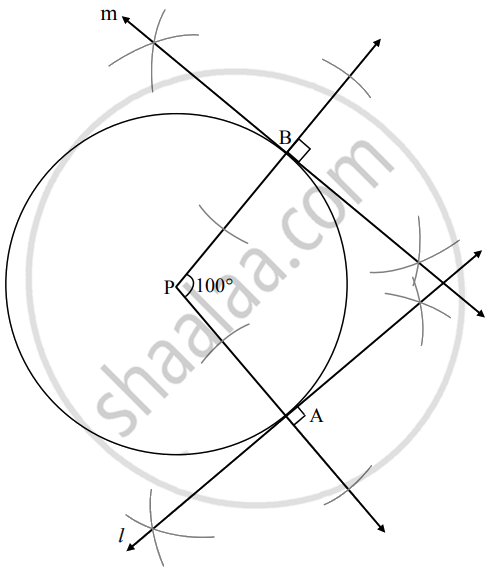# Draw a circle with center P. Draw an arc AB of 100° measure. Perform the following steps to draw tangents to the circle from points A and B. a. Draw a circle with any radius and center P.b. Take - Geometry

Diagram

Draw a circle with center P. Draw an arc AB of 100° measure. Perform the following steps to draw tangents to the circle from points A and B.

1. Draw a circle with any radius and center P.
2. Take any point A on the circle.
3. Draw ray PB such ∠APB = 100°.
4. Draw perpendicular to ray PA from point A.
5. Draw perpendicular to ray PB from point B.

#### SolutionConcept: Construction of a Tangent to the Circle at a Point on the Circle
Is there an error in this question or solution?

Share